# Electromagnetic induction facts for kids

Kids Encyclopedia Facts

Electromagnetic induction is where a voltage or current is produced in a conductor by a changing magnetic flux. It may happen when a magnet is moved in a solenoid, thus changing the magnetic flux.

## Magnetic flux

When a coiled wire is introduced near a magnet, the magnetic lines of force pass through the coil. This causes the magnetic flux to change. Magnetic flux is represented by the symbol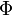${\Phi}$, therefore we can say that${\Phi}$ = BAcos(a) and the resulting unit will be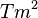$Tm^2$, where T is the unit for magnetic field and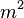$m^2$ is the unit for area.

The changing magnetic flux generates an electromotive force (EMF). This force moves free electrons in a certain way, which constitute a current.

Michael Faraday found that an electromotive force is generated when there is a change in magnetic flux in a conductor.

His laws state that: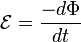$\mathcal{E} = {-{d\Phi} \over dt}$

where,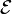$\mathcal{E}$ is the electromotive force, measured in volts;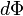${d\Phi}$ is the change in magnetic flux, measured in webers;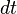$dt$ is the change in time, measured in seconds.

In the case of a solenoid: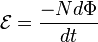$\mathcal{E} = {-N{d\Phi} \over dt}$

where,

N is the number of loops in the solenoid.

## Lenz's law

The negative sign in both equation above is a result of Lenz's law, named after Heinrich Lenz. His law states that the electromotive force (EMF) produces a current that opposes the motion of the changing magnetic flux.

## Images for kidsElectromagnetic induction Facts for Kids. Kiddle Encyclopedia.